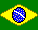Prepositions               | Grammar Index |The most common Portuguese prepositions are contracted as follows when used with the definite article.

 Preposition Definite Article Singular Plural a to/at ao (m) à (f) aos (m) às (f) de of/from do (m) da (f) dos (m) das (f) em in/on no (m) na (f) nos (m) nas (f) por++ by/for pelo (m) pela (f) pelos (m) pelas (f)

++Be careful when using the prepositions para and por.

In English, the indefinite article "a" becomes "an" when it precedes a vowel. In Portuguese, the indefinite articles um (m), and uma (f) must agree with the gender of the accompanying noun. For example, um carro (m) = a car but uma garrafa (f) = a bottle.

While the indefinite article has no plural in English, Portuguese uses the plural forms uns and umas to express the indefinite plural "some." For example, uns carros (m) = some cars and umas garrafas (f) = some bottles.

The prepositions de and em form contractions with the indefinite article as follows:

 Preposition Indefinite Article Singular = "a" Plural = "some" de of/from dum (m) duma (f) duns (m) dumas (f) em in/on num (m) numa (f) nuns (m) numas (f)

Some other important prepositions include:

 antes de before após after depois de after até until/as far as desde since através de through/across sob under sobre over/on por cima de over/above ao lado de beside